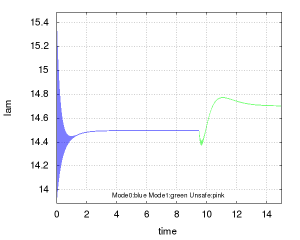# Powertrain Control

The power train control model comes from a suite of benchmarks  that were posed as a challenge problem for the hybrid systems community. Here is a polynomial model with two main modes of the hybrid model.

## Dynamics

### Mode Normal

$$\left \{ \begin{array}{l l } \dot p =& c_1 (2 \bar{\theta} (c_{20} p^2+c_{21} p+c_{22})-c_{12} (c_2+c_3 w p+c_4 w p^2 + c_ 5 w^2 p)) \\ \dot l =& 4 (c_{15}+c_{16} c_{25} ((1/c_{11}) (1+i+c_{13} (c_{24} l-c_{11})) (c_2+c_3 w p_e + c_4 w p_e^2 + c_ 5 p_e w^2 )) \\ &+ c_{17} c_{25}^2 ((1/c_{11}) (1+i+c_{13} (c_{24} l-c_{11})) (c_ 2+c_ 3 w p_e + c_4 w p_e^2 + c_5 p_ e w^2)) \\ &*((1/c_{11}) (1+i+c_{13} (c_{24} l-c_{11})) (c_2+c_3 w p_e + c_4 w p_e^2+c_5 p_e w^2)) \\ &+ c_{18} (c_{12} (c_2+c_3 w p + c_4 w p^2 + c_5 p w^2)) \\&+ c_{19}(c_{12} (c_2+c_3 w p+c_4 w p^2+ c_5 p w^2)) \\&*c_{25}((1/c_{11}) (1+i+c_{13} (c_{24} l-c_{11})) (c_2+c_3 w p_e + c_4 w p_e^2+c_5 p_e w^2))-l) \\ \dot p_e =& c_1 (2 c_{23} \bar{\theta}*(c_{20} p^2+c_{21} p+c_{22})-(c_2+c_3 w p_e+c_4 w p_e^2+c_5 p_e w^2)) \\ \dot i =& c_{14} (c_{24} l – c_{11}) \end{array} \right.$$

Invariant

$$t \geq 9.5~ \&\&~t \leq 20$$

### Mode Startup

$$\left \{ \begin{array}{l l } \dot p =& c_1 (2 \bar{\theta} (c_{20} p^2+c_{21} p+c_{22})-c_{12} (c_2+c_3 w p+c_4 w p^2 + c_ 5 w^2 p)) \\ \dot l =& 4 (c_{15}+c_{16} c_{25} ((1/c_{11}) (c_2+c_3 w p_e + c_4 w p_e^2 + c_ 5 p_e w^2 )) \\ &+ c_{17} c_{25}^2 ((1/c_{11})(c_ 2+c_ 3 w p_e + c_4 w p_e^2 + c_5 p_ e w^2)) \\ &*((1/c_{11})(c_2+c_3 w p_e + c_4 w p_e^2+c_5 p_e w^2)) \\ &+ c_{18} (c_{12} (c_2+c_3 w p + c_4 w p^2 + c_5 p w^2)) \\&+ c_{19}(c_{12} (c_2+c_3 w p+c_4 w p^2+ c_5 p w^2)) c_{25}((1/c_{11})(c_2+c_3 w p_e + c_4 w p_e^2+c_5 p_e w^2))-l) \\ \dot p_e =& c_1 (2 c_{23} \bar{\theta}*(c_{20} p^2+c_{21} p+c_{22})-(c_2+c_3 w p_e+c_4 w p_e^2+c_5 p_e w^2)) \\ \dot i =& 0 \end{array} \right.$$

Invariant

$$t \geq 0 ~ \&\& ~t \leq 9.5$$

## Properties

Please see details in the attached file

## Reachtube generated by C2E2Link to the example file: PowerTrain.hyxml

[1 ]Kapinski, James, Jyotirmoy Deshmukh, Xiaoqing Jin, Hisahiro Ito, and Ken Butts. “Simulation-guided approaches for verification of automotive powertrain control systems.” In American Control Conference (ACC), 2015, pp. 4086-4095. IEEE, 2015.

 Jin, Xiaoqing, Jyotirmoy V. Deshmukh, James Kapinski, Koichi Ueda, and Ken Butts. “Benchmarks for model transformations and conformance checking.” In 1st International Workshop on Applied Verification for Continuous and Hybrid Systems (ARCH). 2014.

 Duggirala, Parasara Sridhar, Chuchu Fan, Sayan Mitra, and Mahesh Viswanathan. “Meeting a Powertrain Verification Challenge.”

 Fan, Chuchu, Parasara Sridhar Duggirala, Sayan Mitra, and Mahesh Viswanathan. “Progress on Powertrain Verification Challenge with C2E2⋆.”